## Graphs in Java

### Introduction

Graphs are a convenient way to store certain types of data. The concept was "stolen" from mathematics and appropriated for the needs of computer science.

There are several ways in which we can describe what graphs are. We will approach graphs first in a highly simplified way, then through trees if the reader is familiar with the concept from earlier experience, and finally as a mathematical term.

### Graphs Simplified

Each graph is consisted of nodes (also called vertices) and edges. We can look at nodes as "chunks of data" and edges as "relations between those chunks of data".

If we want to point out that two chunks of data are related to each other in some way - we'd connect them with an edge. Nodes are usually represented as circles with an identifying mark on them, so that we can distinguish between nodes, and edges are represented via lines (or arrows, as we'll see later) that connect two nodes.

A form of the graph data structure is often used when making maps for a city's bus lines. You'd see a simplified map that shows which stations (nodes) the bus stops at, and the route connecting different stops is what we call an edge: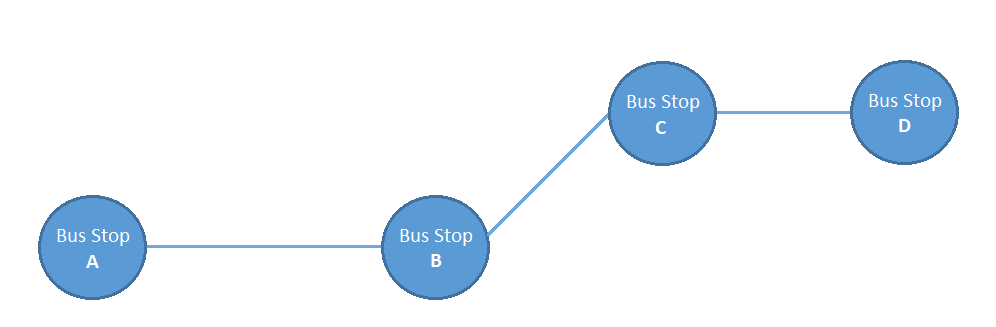This graph shows what different bus stops we have and how they are connected. For example, we can see that, using this bus line, to get from Bus Stop A to Bus Stop C we'd have to go through Bus Stop B - we can't get there any other way.

#### Weighted Graphs

Graphs are very versatile, and that's one of the reasons why they are so popular. Let's say we wanted to add information regarding how long it took on average (in minutes) to get from one bus stop to another. We'd add "weights" to our edges: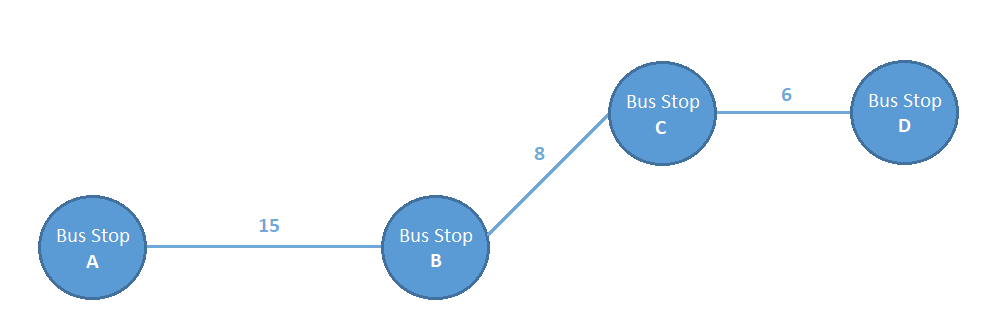Now our graph shows even more information than before, we see how long it takes to get from one stop to another. We can deduce that getting from Bus Stop A to Bus Stop C would take 23 minutes since we'd need to "traverse" the edge connecting Bus Stop A and Bus Stop B that has a weight of 15 and the (Bus Stop B -> Bus Stop C) edge, which has a weight of 8.

The previous example introduced the concept of weighted graphs, i.e. that edges can have values attached to them if we so require.

#### Directed and Undirected Graphs

Now we'll look at another graph concept - directed and undirected graphs. Let's say that our bus line didn't have the same stops in both directions.

More precisely, when our bus starts from Bus Stop A it goes through B, C, and ends at D; however, in the other direction it doesn't go through C but an altogether different bus stop E, before then going through B. We might make something like this: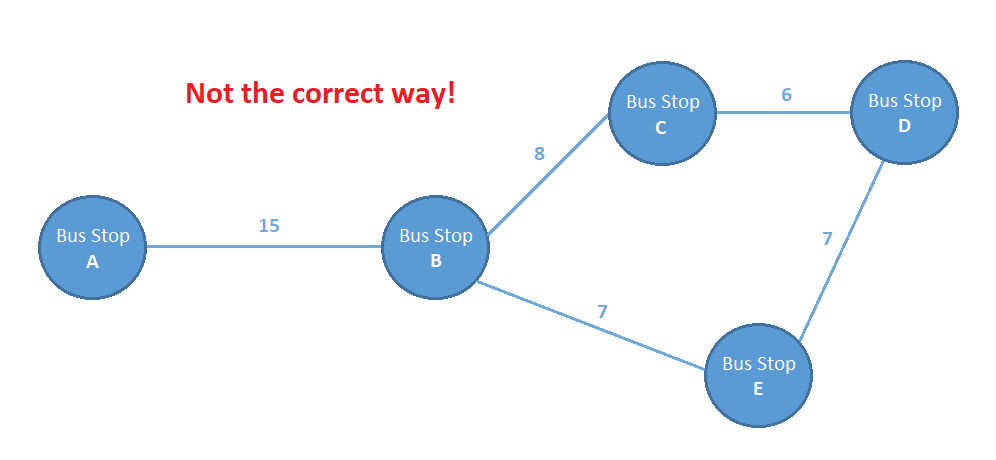As the spoiler in the picture suggests - this approach isn't correct. Just looking at this graph we can't understand which bus stops the bus goes through in which direction, since nothing's stopping us from getting from B to E even though we should only be able to get from E to B.

This is where directed graphs come into play. The edges in directed graphs are represented via arrows instead of lines, an arrow pointing from A to B means "we can get from A to B but not vice versa". If we wanted to say we can get both from A to B and from B to A, we'd use a double-sided arrow.

Now, the cycle is no longer ambiguous: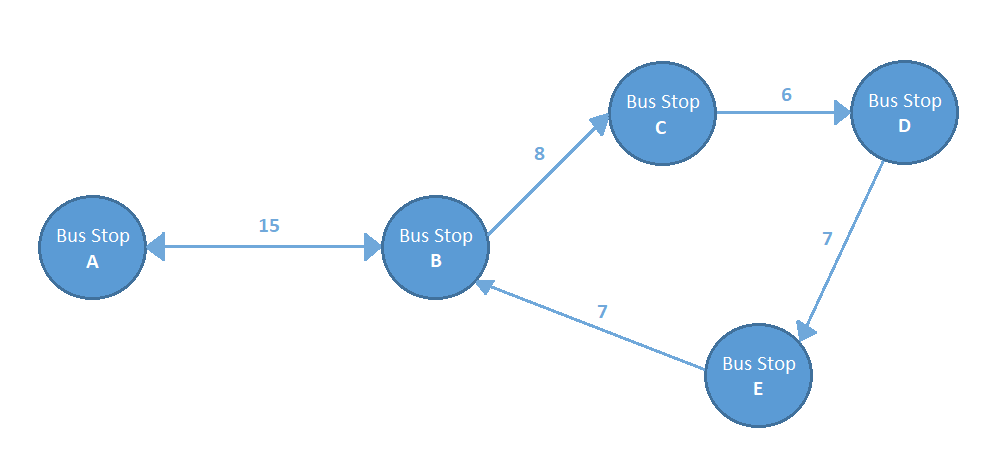Now it's clear that we can't go from B to E or from D to C, etc. It's clear that we can make graphs as simple or as complex as we want to. We could go one step further in our example, if for some reason it took a different amount of time to travel between some bus stops depending on the direction we're going.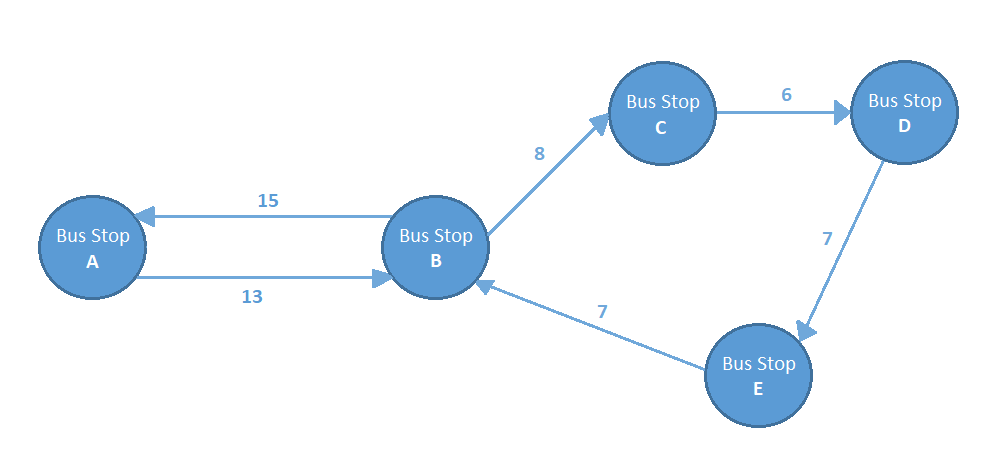### Applications of Graphs

Here are a few examples of data that can be conveniently stored/represented using graphs:

• A set of people and their affiliations: This can be done using an undirected graph, since we're assuming that if person A knows person B, the same is true vice versa. The different people would be represented by nodes, and if person A knows person B there will be an edge connecting the two nodes.
• The structure of a website: Different pages on that website are nodes, and there is a directed edge between page A and page B if a link to page B exists on page A. For example the home page often has links to an About Us and Contact page.
• Mutually dependent tasks: We can represent what tasks need to be completed before task A and what tasks cannot be completed before A has been completed in the following way - if there is a directed edge from B to A, then A cannot be completed before B is completed; if there is a directed edge from A to C, then C cannot be completed before A is completed: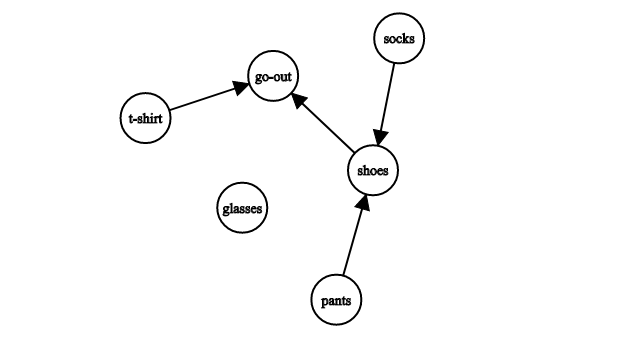Here we can see that we can put on glasses, socks, pants and a t-shirt regardless of other clothes. But we can't put on shoes before putting on pants and socks. And we can't go-out before doing everything else, except putting on glasses, since they might not be necessary. These types of graphs are easily sorted and we can see a feasible order of task execution using Topological Sorting.

Note: A frequent misconception is that graphs need to be connected, or at least that every node needs to be connected to another in some way. This isn't true, and we've demonstrated that in the previous example. A graph can just as easily be consisted of any number of nodes without a single edge between them.

### Graphs Compared to Trees

If you are familiar with trees, graphs are easy to understand - trees are a special kind of graph, i.e. graphs with certain restrictions. Every tree is a graph, but not every graph is a tree.

By definition, trees are undirected graphs that:

• Are acyclic, i.e. we can't traverse back to any starting node we choose without back-tracking
• A cycle is created by adding any edge into the existing tree
• The path between any two nodes is unique
• There is a distinct root node
• The graph is connected (there's a path between every two nodes)

Or in very simplified terms - trees are connected graphs without cycles. For graphs, we just get rid of all these restrictions and keep the nodes and edges concept.

### Graphs as a Mathematical Term

All of the above originated from the mathematical term of a graph. A graph is an ordered pair G = (V, E), where V is a set of vertices and E is the set of edges.

Undirected Graphs: In the case of undirected graph, E has the following definition E ⊆ {{x, y} | (x, y) ∈ V^2}. Sometimes an extra condition is added if we want to avoid edges that have the same start and end point (i.e. x ≠ y).

Directed Graphs: E ⊆ {(x, y) | (x, y) ∈ V^2}, here as well we can add the condition that x ≠ y if we want to avoid edges that start and end in the same node/vertex.

As we can see in the previous definitions of the set of edges E, the only difference is that in the case of directed graphs (x,y) is an ordered pair, while in undirected graphs the pair {x,y} is unordered. This means that in an undirected graph, what matters is that x and y are connected, not in "what order". So we could have written {y,x} instead of {x,y} while we could not have written (y,x) instead of (x,y) for a directed graph.

This all leads us to several important topics when it comes to graph traversal in Java - Representing Graphs in Code, Breadth-First Search, Depth-First Search, and Dijkstra's Algorithm.

### Representing Graphs in Code

Knowing what graphs are, we need a way to represent them in code. This is usually done via Adjacency Lists and Adjacency Matrices. In the following article we present an in-depth look at these implementations in Java:

### Depth-First Search (DFS)

Depth-Frist Search (DFS) searches as far as possible along a branch and then backtracks to search as far as possible in the next branch. This means that in the proceeding Graph, it starts off with the first neighbor, and continues down the line as far as possible.

Breadth First Search (BFS) is a graph-traversal algorithm, often used for finding the shortest path from a starting node to a goal node.

BFS visits "layer-by-layer". This means that it first visits all the children of the starting (root) node. These children are considered as the "second layer", after which their children are visited in the same manner.

### Dijkstra's Algorithm

Dijkstra's Algorithm is a very well-known graph traversal algorithm for finding the shortest path from a given node/vertex to another.

There are several implementations of this algorithm and some even use different data structures and have different applications.

Dijkstra works in a different fashion to the previous algorithms. Instead of starting at the root node, it starts at the goal node and backtracks its way to the starting node.

### DFS vs BFS

A question that most likely came to mind when you were reading about DFS and BFS was:

"What's the practical difference?"

The theory is clear; BFS first visits the nodes closest to our starting node while DFS goes as deep into the graph (as far away from our starting node) as possible before continuing with other nodes close to our starting node.

Let's go through some examples:

• If all you want to do is check whether two nodes are connected at all in a graph that doesn't give you a clear reason to prefer one or the other of the graph traversal algorithms - either one
• If you "know" that your two nodes are close to each other if they're connected at all - for example looking for family members in an acquaintance graph - use BFS
• If you want to count all the possible ways to get from one node to another - DFS
• If you want the shortest path (the path that goes through the fewest number of nodes) from one node to another - BFS
• DFS can also be used for some slightly advanced things, like detecting a cycle in a graph, or for checking for strongly connected components (a mathematical term that means "that every node is reachable from every other node") which can later be used for solving 2-satisfiability problems (2-SAT).

### Conclusion

Graphs are a convenient way to store certain types of data. Each graph is consisted of nodes (also called vertices) and edges. We can look at nodes as "chunks of data" and edges as "relations between those chunks of data".

Graph traversal algorithms such as BFS, DFS, Dijkstra's Algorithm and A* are very commonly used in many branches of data science and machine learning.Hey guys, I want to point out that I don't have any social media to avoid mistakes. If you need any help - post it in the comments :)